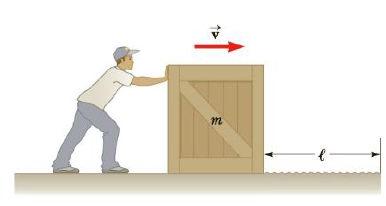Chapter 5, Problem 18P

Chapter
Section
Textbook Problem

A man pushing a crate of mass m = 92.0 kg at a speed of v = 0.850 m/s encounters a rough horizontal surface of length l = 0.65 m as in Figure P5.18. If the coefficient of kinetic friction between the crate and rough surface is 0.558 and he exerts a constant horizontal force of 275 N on the crate, find (a) the magnitude and direction of the net force on the crate while it is on the rough surface, (b) the net work done on the crate while it is on the rough surface, and (c) the speed of the crate when it reaches the end of the rough surface.Figure P5.18

(a)

To determine
The magnitude and direction of the net force on the crate.

Explanation

Given Info:

Mass of the crate is 92.0kg .

Speed of the crate is 0.850m/s .

Length of horizontal rough surface is 0.65m .

Coefficient of kinetic friction between crate and rough surface is 0.358 .

The force excreted by the man on crate is 275N .

In the crate, the normal force acting on the crate is due to the mass of crate. The crate is having the net force acting only on the horizontal direction. The two vertical forces acting on the crate cancels each other.

Formula to calculate the net force on the crate is,

Fnet=Ffk       (I)

• fk is the kinetic friction force
• F is the force excreted on the crate by the man

Since the kinetic friction force is,

fk=μkn=μk(mg)

• μk is the coefficient of kinetic friction
• m is the mass of crate
• g is accele

(b)

To determine
The net work done on the crate while it is on the rough surface.

(c)

To determine
The speed of the crate when it reaches the end of the rough surface.

Still sussing out bartleby?

Check out a sample textbook solution.

See a sample solution

The Solution to Your Study Problems

Bartleby provides explanations to thousands of textbook problems written by our experts, many with advanced degrees!

Get Started